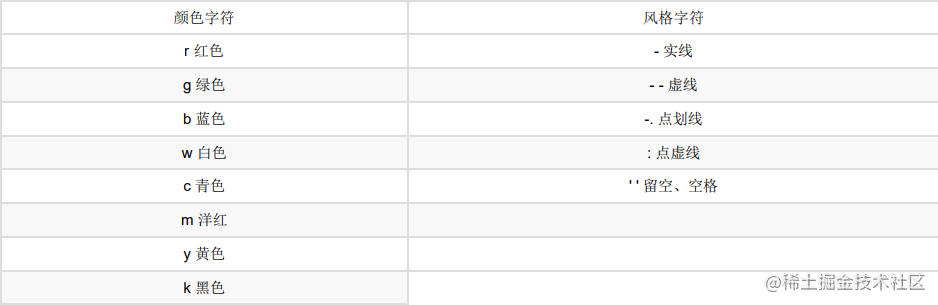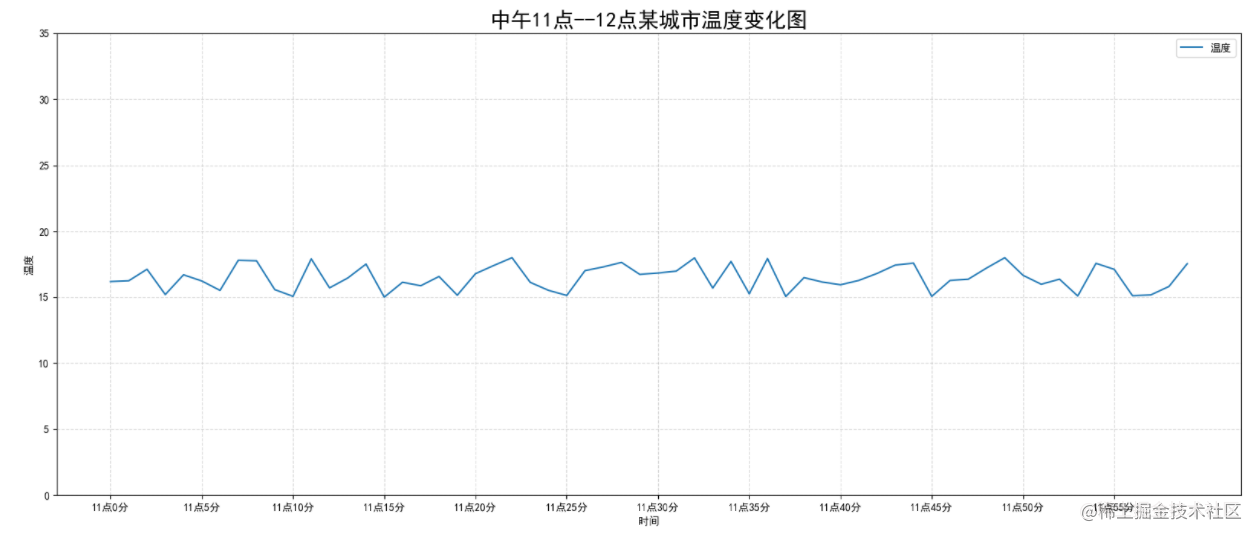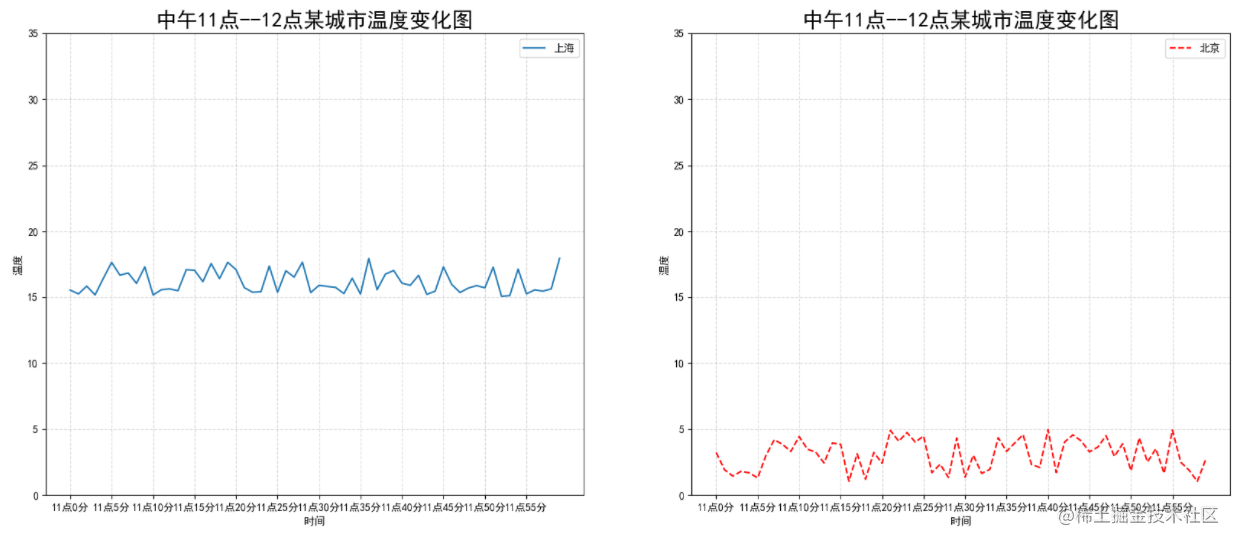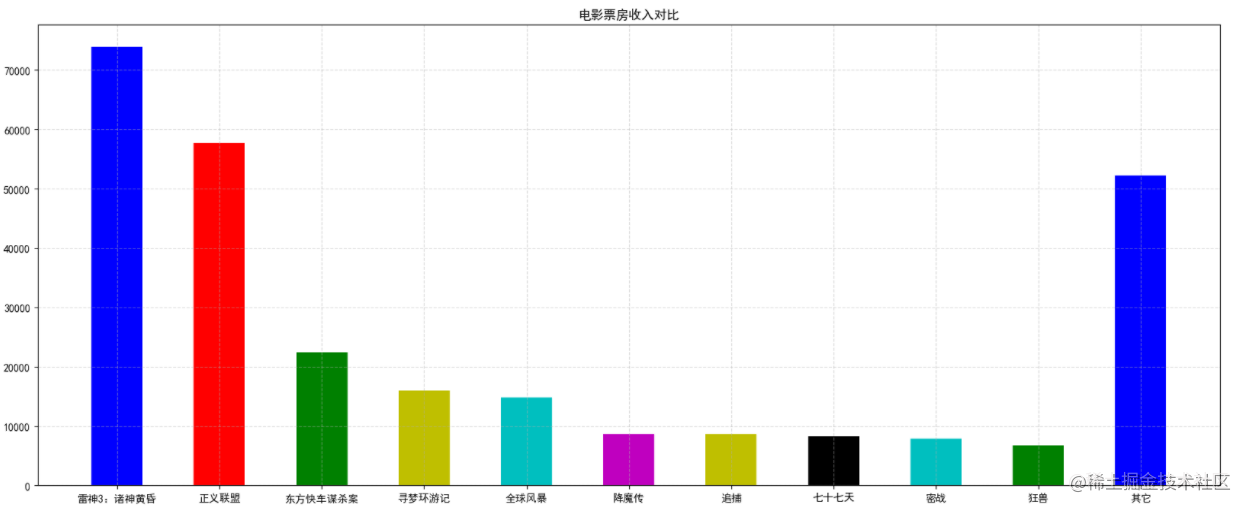# Matplotlib常用繪相簿（機器學習）

## theme: channing-cyan

「這是我參與2022首次更文挑戰的第5天，活動詳情檢視：2022首次更文挑戰

# Matplotlib 繪相簿

## 繪圖流程

```python

import matplotlib.pyplot as plt

plt.figure(figsize=(20,8),dpi=50)#畫板，比例，畫素質量

plt.plot([1,2,3,4,5],[5,8,4,3,5], color='r', linestyle="--") #x,y,顏色,樣式

plt.show()#顯示，顯示之後圖片會被釋放，所以請在顯示之前儲存圖片

```## 美化

```python import matplotlib.pyplot as plt import random from pylab import mpl

# 設定顯示中文字型

mpl.rcParams["font.sans-serif"] = ["SimHei"]

# 設定正常顯示符號

mpl.rcParams["axes.unicode_minus"] = False

# 0.準備資料

x = range(60) y_shanghai = [random.uniform(15, 18) for i in x]

# 1.建立畫布

plt.figure(figsize=(20, 8), dpi=100)

# 2.繪製圖像

plt.plot(x, y_shanghai,label="溫度")

# 構造x,y軸刻度標籤

x_ticks_label = ["11點{}分".format(i) for i in x] y_ticks = range(40)

# 刻度顯示,還可以顯示刻度對應的標籤

plt.xticks(x[::5], x_ticks_label[::5]) plt.yticks(y_ticks[::5])

# 2.2 新增網格顯示

plt.grid(True, linestyle="--", alpha=0.5)

# 2.3 新增描述資訊

plt.xlabel("時間") plt.ylabel("溫度") plt.title("中午11點--12點某城市溫度變化圖", fontsize=20)

# 2.4 影象儲存

plt.savefig("./test.png") plt.legend(loc=0)

# 3.影象顯示

plt.show() ```## 繪製多圖

```python import matplotlib.pyplot as plt import random from pylab import mpl

# 0.準備資料

x = range(60) y_shanghai = [random.uniform(15, 18) for i in x] y_beijing = [random.uniform(1, 5) for i in x]

# plt.figure(figsize=(20, 8), dpi=100)

fig, axes = plt.subplots(nrows=1, ncols=2, figsize=(20, 8), dpi=100)

# plt.plot(x, y_beijing, color="r", linestyle="--", label="北京")

axes.plot(x, y_shanghai, label="上海") axes.plot(x, y_beijing, color="r", linestyle="--", label="北京")

# 構造x,y軸刻度標籤

x_ticks_label = ["11點{}分".format(i) for i in x] y_ticks = range(40)

# plt.yticks(y_ticks[::5])

axes.set_xticks(x[::5]) axes.set_yticks(y_ticks[::5]) axes.set_xticklabels(x_ticks_label[::5]) axes.set_xticks(x[::5]) axes.set_yticks(y_ticks[::5]) axes.set_xticklabels(x_ticks_label[::5])

# plt.grid(True, linestyle="--", alpha=0.5)

axes.grid(True, linestyle="--", alpha=0.5) axes.grid(True, linestyle="--", alpha=0.5)

# plt.title("中午11點--12點某城市溫度變化圖", fontsize=20)

axes.set_xlabel("時間") axes.set_ylabel("溫度") axes.set_title("中午11點--12點某城市溫度變化圖", fontsize=20) axes.set_xlabel("時間") axes.set_ylabel("溫度") axes.set_title("中午11點--12點某城市溫度變化圖", fontsize=20)

# # 2.4 影象儲存

plt.savefig("./test.png")

# plt.legend(loc=0)

axes.legend(loc=0) axes.legend(loc=0)

# 3.影象顯示

plt.show() ```## 繪製不同的影象

### 散點圖

```python

x = [225.98, 247.07, 253.14, 457.85, 241.58, 301.01, 20.67, 288.64, 163.56, 120.06, 207.83, 342.75, 147.9 , 53.06, 224.72, 29.51, 21.61, 483.21, 245.25, 399.25, 343.35] y = [196.63, 203.88, 210.75, 372.74, 202.41, 247.61, 24.9 , 239.34, 140.32, 104.15, 176.84, 288.23, 128.79, 49.64, 191.74, 33.1 , 30.74, 400.02, 205.35, 330.64, 283.45] # 1.建立畫布 plt.figure(figsize=(20, 8), dpi=100) # 2.繪製散點圖 plt.scatter(x, y) # 3.顯示影象 plt.show()

```

### 柱狀圖

```python movie_name = ['雷神3：諸神黃昏','正義聯盟','東方快車謀殺案','尋夢環遊記','全球風暴','降魔傳','追捕','七十七天','密戰','狂獸','其它']

# 橫座標

x = range(len(movie_name))

# 票房資料

y = [73853,57767,22354,15969,14839,8725,8716,8318,7916,6764,52222]

# 1.建立畫布

plt.figure(figsize=(20, 8), dpi=100)

# 2.繪製柱狀圖

plt.bar(x, y, width=0.5, color=['b','r','g','y','c','m','y','k','c','g','b'])

# 2.1b修改x軸的刻度顯示

plt.xticks(x, movie_name)

# 2.2 新增網格顯示

plt.grid(linestyle="--", alpha=0.5)

# 2.3 新增標題

plt.title("電影票房收入對比")

# 3.顯示影象

plt.show()

```## 動態繪圖

### 方案一

```python import matplotlib.pyplot as plt fig, ax = plt.subplots() y1 = [] x1 = [] for i in range(50): x1.append(i) y1.append(i) # 每迭代一次，將i放入y1中畫出來 ax.cla() # 清除鍵 ax.plot(x1,y1)

``````plt.pause(0.1)
``````

```

### 方案二

```python from matplotlib.animation import FuncAnimation```

• fig： 用於顯示動態效果的畫布，即Figure物件；
• func： 函式名，重複呼叫的功能函式；
• frames： 每一幀資料，通常是可迭代物件，依次取出每一個數據傳遞給功能函式；
• init_func： 初始函式，用於執行初始化操作；
• fargs： 傳遞給功能函式的額外引數；
• save_count： 儲存計數，預設為100；
• interval： 重複呼叫功能函式的間隔時間，單位為毫秒，預設為200；
• repeat_delay： 動畫結束後，重複執行動畫的間隔時間，單位為毫秒；
• repeat： 動畫執行結束後，是否重複，預設為True；
• blit： 是否更新所有點，即更新所有點還是僅更新變化的點，預設為False；
• cache_frame_data： 是否快取資料，預設為True；

```python

import numpy as np import matplotlib.pyplot as plt from matplotlib.animation import FuncAnimation

fig = plt.figure(figsize=(10, 5)) # 建立圖 plt.rcParams["font.family"] = "FangSong" # 支援中文顯示 plt.ylim(-12, 12) # Y軸取值範圍 plt.yticks([-12 + 2 * i for i in range(13)], [-12 + 2 * i for i in range(13)]) # Y軸刻度 plt.xlim(0, 2 * np.pi) # X軸取值範圍 plt.xticks([0.5 * i for i in range(14)], [0.5 * i for i in range(14)]) # X軸刻度 plt.title("函式 y = 10 * sin(x) 在[0,2Π]區間的曲線") # 標題 plt.xlabel("X軸") # X軸標籤 plt.ylabel("Y軸") # Y軸標籤 x, y = [], [] # 用於儲存繪圖資料，最開始時什麼都沒有，預設為空

def update(n): # 更新函式 x.append(n) # 新增X軸座標 y.append(10 * np.sin(n)) # 新增Y軸座標 plt.plot(x, y, "r--") # 繪製折線圖

ani = FuncAnimation(fig, update, frames=np.arange(0, 2 * np.pi, 0.1), interval=50, blit=False, repeat=False) # 建立動畫效果 plt.show() # 顯示圖片 ```